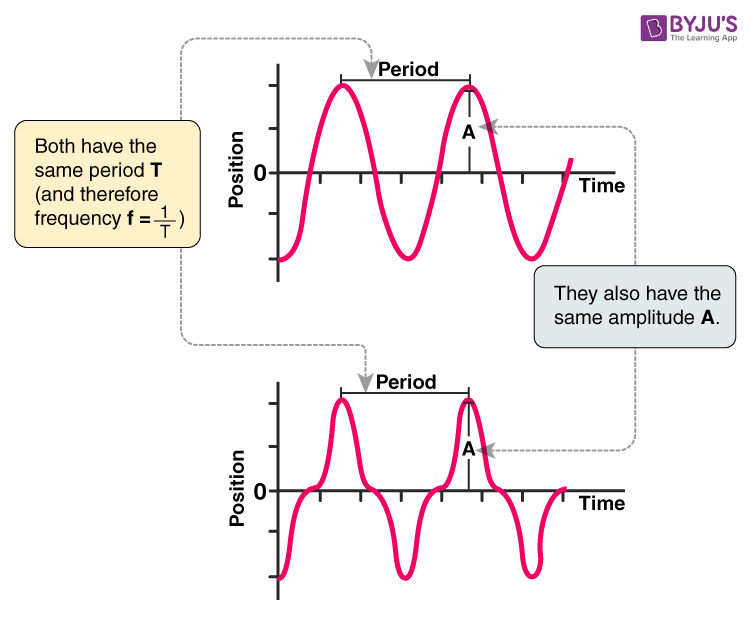# Oscillatory Motion Formula

A motion is said to be oscillatory if it is repetitive in which an object repeats the same movement over and over. In the absence of friction, the body can be in oscillation forever. But in reality, the system settles in the state of equilibrium eventually. Let’s learn the calculation of the frequency of oscillatory motion.

Example: loaded spring, the motion of a pendulum.Here the frequency of the oscillatory motion is calculated by

$$\begin{array}{l}f=\frac{1}{T}\end{array}$$

Where,

f = frequency measured in Hz

one hertz is equal to one oscillation cycle per second

T = time period of motion of waves

For more such formulas on various types of motion, refer BYJU’S app!!